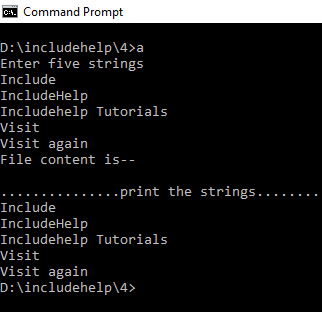# fsetpos() function in C language with Example

Here, we are going to learn about the fsetpos() function of library header stdio.h in C language with its syntax, example.
Submitted by Souvik Saha, on January 24, 2019

## fsetpos() function in C

Prototype:

```    int fsetpos(FILE* filename, fpos_t *position);
```

Parameters:

```    FILE* filename, fpos_t *position
```

Return type: int

Use of function:

In file handling, through the fsetpos() function we set the position of the input file stream indicator at the point which we get from fgetpos(). Whenever we need to fix the file indicator position in the file, we need to use the function fgetpos(). The prototype of the fgetpos() function is:

```    int fsetpos(FILE* filename, fpos_t *position);
```

Here, the data type of the position variable must be fpos_t type. A return value of zero means the successful operation and non-zero returns means failure.

## fsetpos() example in C

```#include <stdio.h>
#include <stdlib.h>

int main()
{
//Initialize the file pointer
FILE* f;
//Take a array of characters
char ch;
//Initialize the position variable
fpos_t pos;

//Create the file for write operation
f = fopen("includehelp.txt", "w+");
//Store the value of the function point indicator
fgetpos(f, &pos);
printf("Enter five strings\n");
for (int i = 0; i < 4; i++) {
//take the strings from the users
scanf("%[^\n]", &ch);
//write back to the file
fputs(ch, f);
//every time take a new line for the new entry string
//except for last entry.Otherwise print the last line twice
fputs("\n", f);
//clear the stdin stream buffer
//if we don't write this then after taking string
//%[^\n] is waiting for the '\n' or white space
fflush(stdin);
}
//take the strings from the users
scanf("%[^\n]", &ch);
fputs(ch, f);
//set the indicator position to the initial position of the file
fsetpos(f, &pos);
printf("\n...............print the strings..............\n\n");
while (!feof(f)) {
//takes the first 100 character in the character array
fgets(ch, 100, f);
//and print the strings
printf("%s", ch);
}

//close the file
fclose(f);

return 0;
}
```

Output